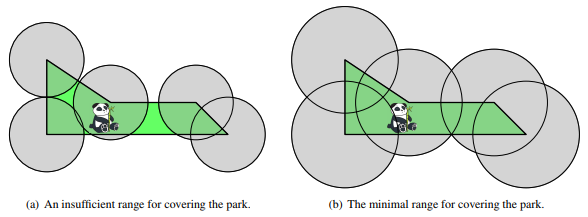November 08, 2023

1. 给定 $x,c,d$，添加一个编号为 $n+1$ 颜色为 $c$ 的节点，向点 $x$ 连一条长度为 $d$ 的边
2. 给定 $x,c$，将点 $x$ 的颜色变成 $c$

$1\le q\le 5 \times 10^5$

January 21, 2021

1. 有两个空序列 $A$$B$
2. 枚举 $S_i=1$ 的每个 $i$：如果 $P_i$ 是偶数，则将其放到 $A$ 的末尾；否则放到 $B$ 的末尾；
3. 如果 $t=0$ 则令 $C=\overline{AB}$，否则令 $C=\overline{BA}$
4. 枚举 $S_i=1$ 的每个 $i$：将 $P_i$ 替换为 $C$ 的开头元素，删去 $C$ 的开头元素。

$1\le n\le 15000$

December 17, 2020

$n,q\le6\times10^5,\ 1\leq a_i < x\leq 10^9$

December 16, 2020

$n\le 10^5$

October 18, 2020$3 \leq n \leq 2000,\ -10^4 \leq x_i,y_i \leq 10^4$

October 10, 2020

1. $x,y$，将 $x$ 位置的值修改为 $y$
2. $l,r,x$，查询区间$[l,r]$中有多少子区间的最大值小于或等于 $x$

$n,m \leq 3 \times 10^5$

October 04, 2020

September 17, 2020

• $b,e < 2^{64}$，且是正整数
• 对于所有 $0 \leq i < n$$a+id$ 的十进制表示是 $F_{b+ie}$ 的十进制表示的后 $18$ 位的子串（如果没有 $18$ 位自动补前导零）。其中 $F_i$ 是指斐波那契数列的第 $i$ 项。

$1 \leq a+(n-1)d \leq 10^6$

September 15, 2020

$1 \leq a_i, n \leq 60$，保证 $a_i$ 两两不同。

August 31, 2020

$n, w_i \leq 1000$